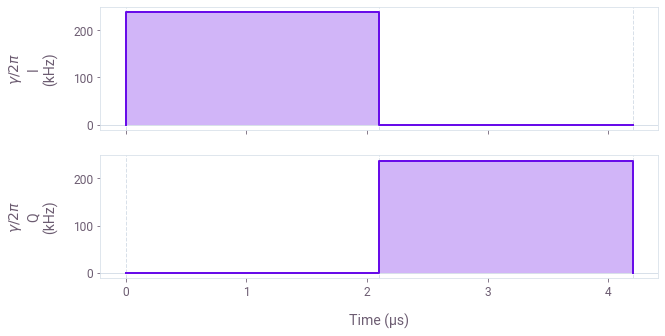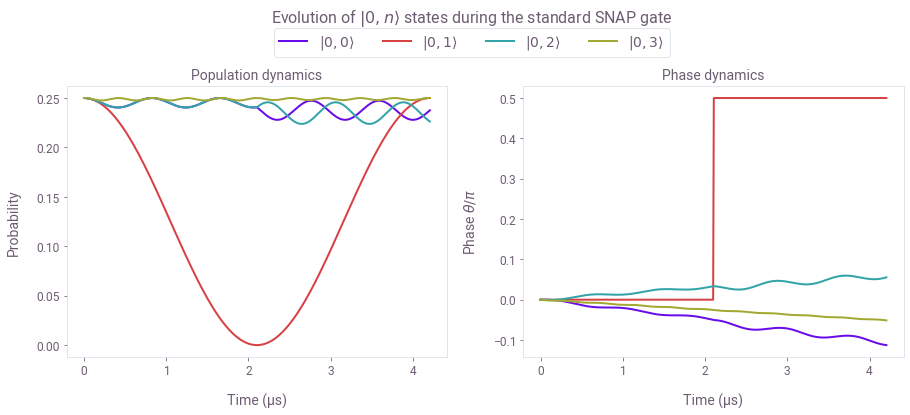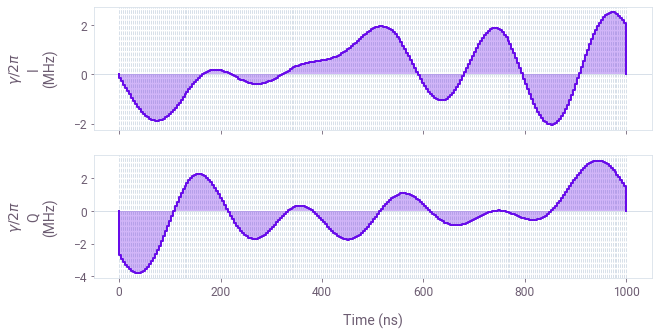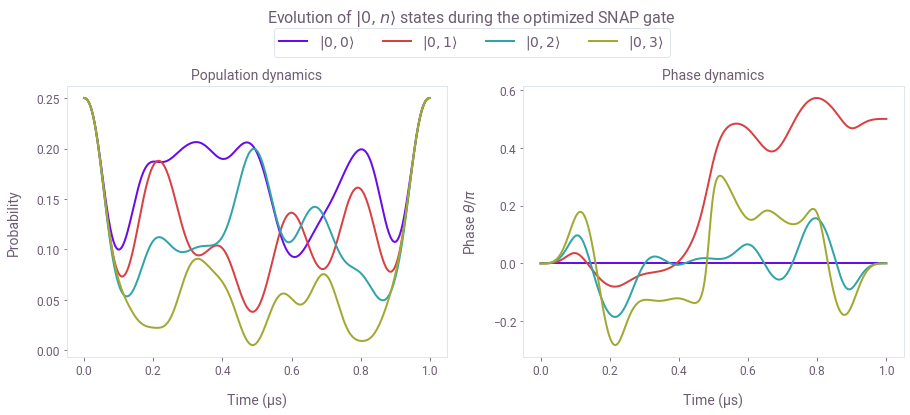Designing fast optimal SNAP gates in superconducting resonators

Engineering fast, leakage-free gates in superconducting cavity-qubit systems

Boulder Opal provides a flexible toolkit for control optimization in high-dimensional Hilbert spaces. The exceptional size of the Hilbert space of quantum oscillators can be viewed as a resource to encode and process quantum information, but can also introduce channels for gate-performance degradation. For instance, leakage to unwanted levels due to the spectral content of a control waveform can arise as the number of relevant oscillator modes increases. In practice, long pulse durations are used to narrow the operation's spectral range and, consequently, prevent leakage to neighboring levels. Longer gates, however, expose the system to other limiting factors such as spontaneous decay and cavity losses.

In this notebook, you'll have the opportunity to use Boulder Opal optimization tools to make Selective Number-dependent Arbitrary Phase (SNAP) gates with transmons faster while suppressing leakage at the same time. We will cover:

• Simulating leakage in SNAP gates
• Optimizing fast SNAP gates which suppress leakage to a ladder of states in a harmonic oscillator
• Validating optimized gate performance using simulation

Ultimately we will show how model-based numeric optimization provides a means to dramatically enhance gate speed without performance degradation in qubit-oscillator systems.

Imports and initialization

import matplotlib.pyplot as plt
import numpy as np
from qctrlvisualizer import get_qctrl_style, plot_controls
from scipy.linalg import expm

# Q-CTRL imports
from qctrl import Qctrl

# Starting a session with the API
qctrl = Qctrl()

# Plotting style
plt.style.use(get_qctrl_style())

# Data containers
controls = {}
simulation_results = {}

Evaluating a standard SNAP gate

The system consists of a superconducting transmon system coupled to a cavity in the dispersive limit, as given by the following Hamiltonian:

$$H = \omega_C a^\dagger a + \frac{K}{2} (a^\dagger)^2 a^2 + \omega_T b^\dagger b + \chi a^\dagger a b^\dagger b,$$

where $\omega_C$ is the cavity transition frequency, $a$ is the annihilation operator of a cavity excitation, $b$ is the annihilation operator of the transmon system, $K$ is the Kerr coefficient, $\omega_T$ is the transmon frequency, and $\chi$ is the dispersive shift. The basis states in the Hilbert space will be denoted by $|i,j\rangle =|i\rangle_T \otimes |j\rangle_C$, for the transmon number state $|i\rangle_T$ and cavity number state $|j\rangle_C$.

For a complex drive $\gamma(t)= I(t) + i Q(t)$ applied to the transmon with frequency $\omega_D$, the Hamiltonian can be written in the interaction picture with respect to $\omega_C a^\dagger a + \omega_D b^\dagger b$:

$$H_I = \frac{K}{2} (a^\dagger)^2 a^2 + \delta_T b^\dagger b + \chi a^\dagger a b^\dagger b + \left(\gamma (t) b + H.c.\right),$$

where $\delta_T = \omega_T - \omega_D$.

Only the lowest two energy levels of the transmon system are treated in this notebook, such that there is no transmon anharmonicity term in $H_I$ to characterize higher-energy transmon states. Note that in this configuration, the transmon qubit will exhibit a spectrum of transition frequencies, set $\chi$ apart, each corresponding to a different excitation state of the cavity.

The objective of a SNAP gate is to impart a phase of $\theta$ to a target Fock state $j$ of the cavity $|j\rangle_C \rightarrow e^{i\theta} |j\rangle_C$. The standard implementation of SNAP gates consists of two control $\pi$ pulses applied on the transmon qubit based on a target Fock state of the cavity. The pulses are performed around different axes, offset apart by an angle $\theta$. By the end of the gate, the qubit makes a net $2\pi$ rotation, disentangling from the targeted Fock state while imparting the desired phase $\theta$ to it.

Obtaining a standard SNAP gate

To set up the system to evaluate a SNAP gate, we first define the basic parameters and target operation. Note that the transmon drive detuning is determined by the target cavity Fock state, to resolve a particular transition frequency.

K = 2 * np.pi * 4e3  # rad.Hz
chi = 2 * np.pi * 1.189e6  # rad.Hz

# SNAP gate parameters
target_Fock_n = 1  # Fock state that the SNAP gate is targeting
theta = np.pi / 2  # SNAP gate angle
delta_T = -chi * target_Fock_n
rabi_rate = 0.2 * chi

Next we generate the standard SNAP drive control.

segment_duration = np.pi / rabi_rate
controls["Standard"] = {
"$\\gamma$": [
{"duration": segment_duration, "value": rabi_rate},
{
"duration": segment_duration,
"value": np.exp(1j * np.pi - 1j * theta) * rabi_rate,
},
]
}
plot_controls(plt.figure(), controls["Standard"], polar=False)The plots display the drive pulses $\gamma(t)$ applied on the transmon qubit. Note that since the standard SNAP gates demand high spectral selectivity, the qubit Rabi rates need to be smaller than the the qubit-cavity coupling $|\gamma| < \chi$, making the gate relatively long as in Heeres et al.

Simulating the system evolution under the standard controls

We can now simulate the evolution of the system. For this purpose, the first four states of the cavity ($n_c=4$) will be evenly populated while the qubit will be initialized in the ground state $| 0\rangle_T$, making the total initial state: $(|0, 0\rangle + |0, 1\rangle + |0, 2\rangle + |0, 3\rangle)/2$.

We target the first Fock state $|1\rangle_C$ with the SNAP gate, evolving the initial state into $(|0, 0\rangle + e^{i\theta}|0, 1\rangle + |0, 2\rangle + |0, 3\rangle)/2$, for the gate angle $\theta$, here chosen to be $\theta=\pi/2$.

First, we set up the simulation parameters, initial state, and operators.

# Simulation dimensions for the transmon and cavity
sim_dimt = 2
sim_dimc = 4

# Gate parameters
drive_control_segments = controls["Standard"]["$\gamma$"]
gate_duration = np.sum([s["duration"] for s in drive_control_segments])
sample_points = 512  # Number of evolution samples
sample_times = np.linspace(0, gate_duration, sample_points)

# Set up initial state
c_initial_state = np.full((sim_dimc), 1)
c_initial_state = c_initial_state / np.sqrt(
np.abs(c_initial_state.dot(np.conj(c_initial_state)))
)
t_initial_state = np.zeros(sim_dimt)
t_initial_state = 1
initial_state = np.kron([t_initial_state], [c_initial_state]).T

# Set up basic operators and Hamiltonian terms
a = np.diag(np.sqrt(np.arange(1, sim_dimc)), k=1)
b = np.diag(np.sqrt(np.arange(1, sim_dimt)), k=1)
Hosc = K / 2 * np.kron(np.eye(sim_dimt), np.dot(np.dot(a.T, a.T), np.dot(a, a)))
Htrans = delta_T * np.kron(np.dot(b.T, b), np.eye(sim_dimc))
Hint = chi * np.kron(np.dot(b.T, b), np.dot(a.T, a))

# Set up drive
control_T = np.kron(b.T, np.eye(sim_dimc))
control_T_values = np.array([s["value"] for s in drive_control_segments])

Next, we perform the simulation and return the states at the specified sample_times.

graph = qctrl.create_graph()

# Build the time-dependent Hamiltonian terms
driveT_signal = graph.pwc_signal(values=control_T_values, duration=gate_duration)
H_drive_T = graph.pwc_operator_hermitian_part(driveT_signal * control_T)

# Construct the total Hamiltonian
hamiltonian = Hosc + Htrans + Hint + H_drive_T

# Calculate states over time
unitaries = graph.time_evolution_operators_pwc(
hamiltonian=hamiltonian, sample_times=sample_times
)
states = unitaries @ initial_state
states.name = "states"

simulation_results["Standard"] = qctrl.functions.calculate_graph(
graph=graph, output_node_names=["states"]
)

To understand the evolution of the cavity states under the standard SNAP gate, it's instructive to plot both the probabilities and the phases of all the $|0,n\rangle$ states. In the below cell, we extract these values.

simulation_result = simulation_results["Standard"].output["states"]["value"]

# reshape states into [time, transmon, cavity]
# and take the probability coefficients for the transmon ground state
ground_states = np.reshape(simulation_result, [512, 2, 4])[:, 0, :]

# calculate populations and phases
populations = np.abs(ground_states) ** 2
phases = np.angle(ground_states)

Now we can plot the dynamics.

fig, axs = plt.subplots(1, 2, figsize=(15, 5))
fig.suptitle(r"Evolution of $|0,n\rangle$ states during the standard SNAP gate", y=1.1)

ax = axs
ax.set_xlabel("Time (µs)")
ax.set_ylabel(r"Probability")
ax.set_title(r"Population dynamics")
ax.plot(sample_times / 1e-6, populations)

ax = axs
ax.set_xlabel("Time (µs)")
ax.set_ylabel(r"Phase $\theta/\pi$")
ax.set_title(r"Phase dynamics")
ax.plot(sample_times / 1e-6, phases / np.pi)

labels = [rf"$|0,{n}\rangle$" for n in range(4)]
fig.legend(labels=labels, loc="center", bbox_to_anchor=(0.5, 1.0), ncol=4)

plt.show()Here we present the evolution of population (left) and relative phase (right) of the $|0,n\rangle$ states under the SNAP gate targeting $|1\rangle_C$ Fock state. The standard controls induce significant leakage into the neighboring states the which can only be contained by significantly extending the gate duration. Observe that the phase of $|0,1\rangle$ changes from 0 to $\pi/2$ in line with the offset between the two $\pi$-pulse frames, however the phases of neighboring Fock states suffer from a nontrivial combination of leakage and Kerr effect.

Creating Q-CTRL fast SNAP gates optimized for leakage suppression

We now use the optimization engine in Boulder Opal to obtain control pulses that perform a faster SNAP while minimizing leakage to other Fock states in the cavity. In this case, the unitary target operation will be $e^{i\theta}|0,1\rangle\langle 0,1|$ projected onto the $|0\rangle\langle 0|_T \otimes I_C$ subspace since the final population of the qubit's excited state will be zero by the end of the gate.

Generating a control pulse

To optimize a control pulse, we first specify optimization parameters such as the number of pulse segments and the target operation, as described in the Design robust single qubit gates using computational graphs tutorial. We obtain smooth control pulses by applying a sinc filter with a specified cutoff frequency: here the the maximum Rabi rate is also used as the cutoff frequency for the control. For more information, see the How to add smoothing and band-limits to optimized controls user guide.

# Optimization parameters
dimt = 2
dimc = 4
max_rabi_rate_T = 4.5 * chi
cutoff_frequency = max_rabi_rate_T  # Sinc filter cutoff frequency
gate_duration = 1e-6  # s
number_of_optimizer_vars = 64
number_of_segments = 256  # Smooth control segments
optimization_count = 20  # Number of optimization runs

# Set up target operation in the appropriate subspace
SNAP_cav_target_state = np.zeros(dimc)
SNAP_cav_target_state[target_Fock_n] = 1.0
cav_target_operation = expm(
1j
* theta
* np.dot(SNAP_cav_target_state[None, :].T, [SNAP_cav_target_state]).astype(complex)
)
full_target_operation = np.kron(np.eye(dimt), cav_target_operation).astype(complex)
cavity_subspace_projector = np.diag(np.kron(np.array([1.0, 0.0]), np.ones(dimc)))
subspace_target = np.matmul(full_target_operation, cavity_subspace_projector)

# Set up basic operators and Hamiltonian terms
a = np.diag(np.sqrt(np.arange(1, dimc)), k=1)
b = np.diag(np.sqrt(np.arange(1, dimt)), k=1)
H_osc = K / 2 * np.kron(np.eye(dimt), np.dot(np.dot(a.T, a.T), np.dot(a, a)))
H_trans = delta_T * np.kron(np.dot(b.T, b), np.eye(dimc))
H_int = chi * np.kron(np.dot(b.T, b), np.dot(a.T, a))
control_T = np.kron(b.T, np.eye(dimc))

Next, we perform the optimization.

graph = qctrl.create_graph()

# Set up a sinc kernel to bandlimit the pulses (if desired)
sinc_kernel = graph.sinc_convolution_kernel(cutoff_frequency)

# Set up optimizer variables for the I component of the transmon drive
drive_iT_vars = graph.optimization_variable(
count=number_of_optimizer_vars,
lower_bound=-max_rabi_rate_T,
upper_bound=max_rabi_rate_T,
)
# Build the pulse signal with segments based on the optimizer variables
drive_iT_raw = graph.pwc_signal(values=drive_iT_vars, duration=gate_duration)

# Apply the filter to the raw pulse
drive_iT_filtered = graph.convolve_pwc(pwc=drive_iT_raw, kernel=sinc_kernel)
# Discretize the filtered drive into the desired number of segments

drive_iT_signal = graph.discretize_stf(
stf=drive_iT_filtered, duration=gate_duration, segment_count=number_of_segments
)

# Set up the Q component of the drive in a similar way
drive_qT_vars = graph.optimization_variable(
count=number_of_optimizer_vars,
lower_bound=-max_rabi_rate_T,
upper_bound=max_rabi_rate_T,
)
drive_qT_raw = graph.pwc_signal(values=drive_qT_vars, duration=gate_duration)
drive_qT_filtered = graph.convolve_pwc(pwc=drive_qT_raw, kernel=sinc_kernel)
drive_qT_signal = graph.discretize_stf(
stf=drive_qT_filtered, duration=gate_duration, segment_count=number_of_segments
)

# Combine the I and Q components
driveT_signal = drive_iT_signal + 1j * drive_qT_signal
driveT_signal.name = "$\gamma$"

# Construct the Hamiltonian
H_drive_T = graph.pwc_operator_hermitian_part(driveT_signal * control_T)
hamiltonian = H_osc + H_trans + H_int + H_drive_T
noise_list = []

# Gate infidelity cost
cost = graph.infidelity_pwc(
hamiltonian=hamiltonian,
target=graph.target(subspace_target),
noise_operators=noise_list,
name="cost",
)

result = qctrl.functions.calculate_optimization(
graph=graph,
cost_node_name="cost",
output_node_names=["$\gamma$"],
optimization_count=optimization_count,
)

print("Cost: " + str(result.cost))
controls["Q-CTRL"] = result.output

plot_controls(plt.figure(), controls["Q-CTRL"], polar=False)
Your task calculate_optimization (action_id="619044") has started. You can use the qctrl.get_result method to retrieve previous results.
Cost: 3.58064689010007e-11The plot above displays the pulses for the optimized SNAP gate. The maximum Rabi rate and cutoff frequency of these controls is set sufficiently high to ensure that all of the populated cavity levels can be simultaneously addressed.

State leakage analysis using simulation

Here we simulate the evolution of the system under the optimized SNAP gate. We again set up the simulation parameters, using the same initial state.

# Gate parameters
drive_control_segments = controls["Q-CTRL"]["$\gamma$"]
gate_duration = np.sum([s["duration"] for s in drive_control_segments])
sample_points = 512
sample_times = np.linspace(0, gate_duration, sample_points)

# Set up initial state
c_initial_state = np.full((sim_dimc), 1)
c_initial_state = c_initial_state / np.sqrt(
np.abs(c_initial_state.dot(np.conj(c_initial_state)))
)
t_initial_state = np.zeros(sim_dimt)
t_initial_state = 1
initial_state = np.kron([t_initial_state], [c_initial_state]).T

# Set up drive
control_T = np.kron(b.T, np.eye(sim_dimc))
control_T_values = np.array([s["value"] for s in drive_control_segments])

Now we can simulate the optimized gate.

graph = qctrl.create_graph()

# Construct the Hamiltonian
driveT_signal = graph.pwc_signal(values=control_T_values, duration=gate_duration)
H_drive_T = graph.pwc_operator_hermitian_part(driveT_signal * control_T)
hamiltonian = Hosc + Htrans + Hint + H_drive_T

# Calculate states over time
unitaries = graph.time_evolution_operators_pwc(
hamiltonian=hamiltonian, sample_times=sample_times
)
states = unitaries @ initial_state
states.name = "states"

simulation_results["Q-CTRL"] = qctrl.functions.calculate_graph(
graph=graph,
output_node_names=["states"],
)

To observe the dynamics, we extract the cavity number-state populations and phases.

simulation_result = simulation_results["Q-CTRL"].output["states"]["value"]

# reshape states into [time, transmon, cavity]
# and take the probability coefficients for the transmon ground state
ground_states = np.reshape(simulation_result, [512, 2, 4])[:, 0, :]

# calculate populations and phases
populations = np.abs(ground_states) ** 2
phases = np.angle(ground_states)

Next, we plot the state evolution for the optimized control.

fig, axs = plt.subplots(1, 2, figsize=(15, 5))
fig.suptitle(r"Evolution of $|0,n\rangle$ states during the optimized SNAP gate", y=1.1)

ax = axs
ax.set_xlabel("Time (µs)")
ax.set_ylabel("Probability")
ax.set_title("Population dynamics")
ax.plot(sample_times / 1e-6, populations)

ax = axs
ax.set_xlabel("Time (µs)")
ax.set_ylabel(r"Phase $\theta/\pi$")
ax.set_title("Phase dynamics")
ax.plot(sample_times / 1e-6, (phases - phases[:, :1]) / np.pi)

labels = [rf"$|0,{n}\rangle$" for n in range(4)]
fig.legend(labels=labels, loc="center", bbox_to_anchor=(0.5, 1.0), ncol=4)

plt.show()Plotted are the populations (left) and relative phases (right) of the $|0,n\rangle$ states during the optimized SNAP gate. The control solution generated using Boulder Opal is shorter than the standard gate because it allowed us to leverage a higher Rabi rate. The impact of leakage is actively controlled throughout the gate, ensuring that the neighboring Fock state populations follow their individual trajectories back to the original starting point. Observe that the relative phases of the states evolve in such a way that the phase of the targeted $|1\rangle_C$ state reaches the specified target value $\theta =\pi/2$ by the end of the gate, while phases of the neighboring states converge to 0, in the process also eliminating the effects of the Kerr term.